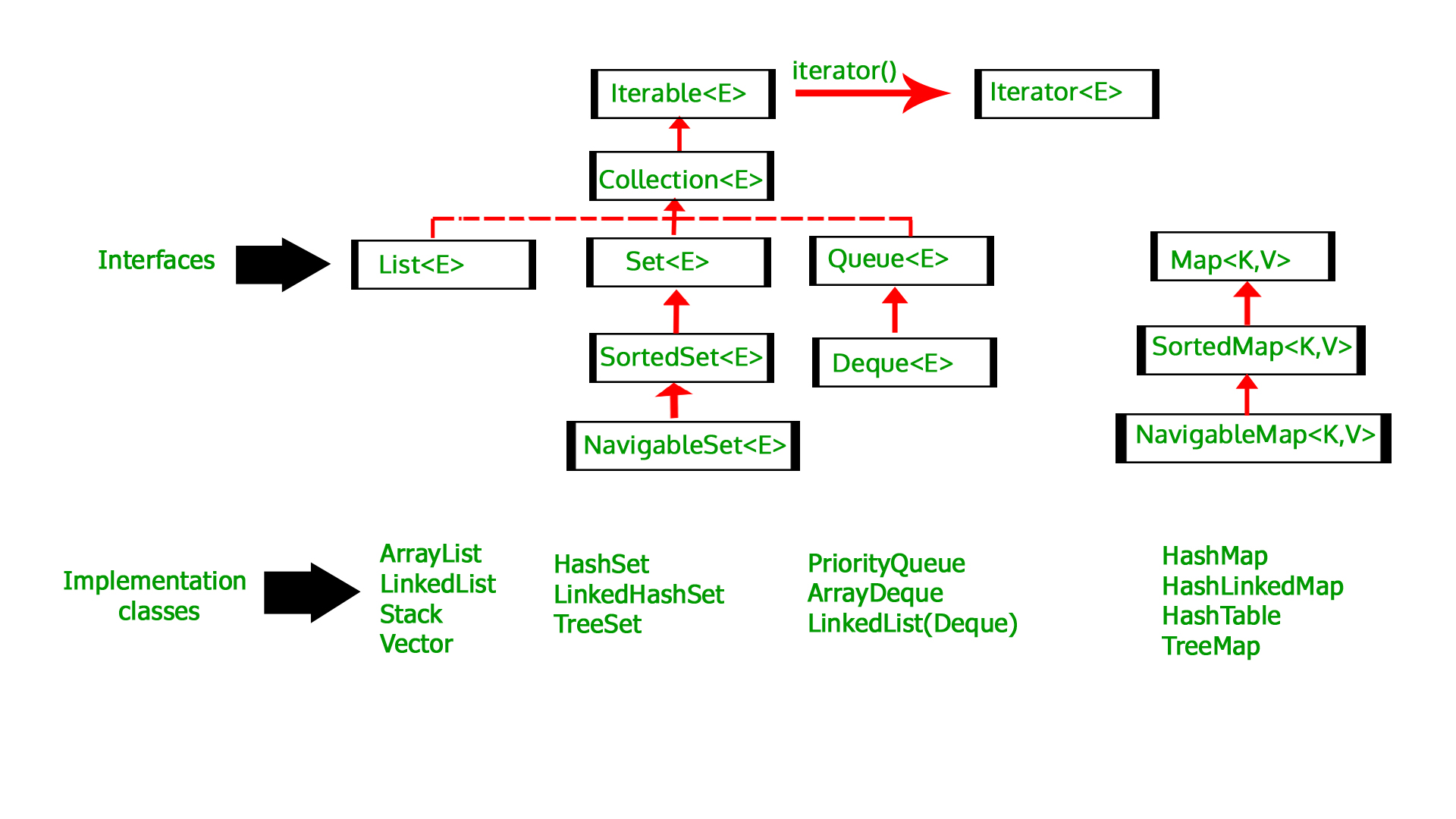# Finding minimum and maximum element of a Collection in Java

• Difficulty Level : Medium
• Last Updated : 22 Nov, 2018

A Collection is a group of individual objects represented as a single unit. Java provides Collection Framework which defines several classes and interfaces to represent a group of objects as a single unit. These are:Finding minimum and maximum element of a Collection can be easily done using the Collections.min() and Collections.max() method. These are static method of Collections Class in Java. Hence they can be accessed directly with the help of class name as shown below.

```Object ob = Collections.min(Collection<E> c);
Object ob = Collections.max(Collection<E> c);
```

As seen in the syntax above, the Collections.min() and Collections.max() methods take the Collection as parameter. This is the Collection of which max/min element is to be found. Hence any class implementing the Collection interface can be passed as the parameter in these functions, such as Arrays, LinkedList, ArrayList, Set, etc. And therefore the maximum and minimum element of any collection can be easily found.

Below examples demonstrate the procedure to find the minimum and maximum element of some of the collections.

Example 1: In List

 `// Java program to find the minimum and``// maximum element in a List`` ` `import` `java.util.*;`` ` `public` `class` `GFG {``    ``public` `static` `void` `main(String args[]) ``throws` `Exception``    ``{`` ` `        ``// Get the ArrayList``        ``List list = ``new` `ArrayList();`` ` `        ``// populate the list``        ``list.add(``12``);``        ``list.add(``53``);``        ``list.add(``30``);``        ``list.add(``8``);`` ` `        ``// printing the List``        ``System.out.println(``"List: "` `+ list);`` ` `        ``// getting minimum value``        ``// using min() method``        ``int` `minList = Collections.min(list);`` ` `        ``// getting maximum value``        ``// using max() method``        ``int` `maxList = Collections.max(list);`` ` `        ``// printing the minimum value``        ``System.out.println(``"Minimum value of list is: "``                           ``+ minList);`` ` `        ``// printing the maximum value``        ``System.out.println(``"Maximum value of list is: "``                           ``+ maxList);``    ``}``}`

Output:

```List: [12, 53, 30, 8]
Minimum value of list is: 8
Maximum value of list is: 53
```

Example 2: In Set

 `// Java program to find the minimum and``// maximum element in a Set`` ` `import` `java.util.*;`` ` `public` `class` `GFG {``    ``public` `static` `void` `main(String args[]) ``throws` `Exception``    ``{`` ` `        ``// Get the HashSet``        ``Set set = ``new` `HashSet();`` ` `        ``// fill the hashSet``        ``set.add(``3``);``        ``set.add(``6``);``        ``set.add(``2``);``        ``set.add(``9``);`` ` `        ``// printing the Set``        ``System.out.println(``"Set: "` `+ set);`` ` `        ``// getting minimum value``        ``// using min() method``        ``int` `minSet = Collections.min(set);`` ` `        ``// getting maximum value``        ``// using max() method``        ``int` `maxSet = Collections.max(set);`` ` `        ``// printing the minimum value``        ``System.out.println(``"Minimum value of set is: "``                           ``+ minSet);`` ` `        ``// printing the maximum value``        ``System.out.println(``"Maximum value of set is: "``                           ``+ maxSet);``    ``}``}`

Output:

```Set: [2, 3, 6, 9]
Minimum value of set is: 2
Maximum value of set is: 9
```

Example 3: In Arrays

 `// Java program to find the minimum and``// maximum element in an Array`` ` `import` `java.util.*;`` ` `public` `class` `GFG {``    ``public` `static` `void` `main(String args[]) ``throws` `Exception``    ``{`` ` `        ``// Get the Array``        ``Integer arr[] = { ``2``, ``5``, ``1``, ``8``, ``34``, ``20``, ``4` `};`` ` `        ``// printing the Array``        ``System.out.println(``"Array: "` `+ Arrays.toString(arr));`` ` `        ``// getting minimum value``        ``// using min() method``        ``int` `minArray = Collections.min(Arrays.asList(arr));`` ` `        ``// getting maximum value``        ``// using max() method``        ``int` `maxArray``            ``= Collections.max(Arrays.asList(arr));`` ` `        ``// printing the minimum value``        ``System.out.println(``"Minimum value of Array is: "``                           ``+ minArray);`` ` `        ``// printing the maximum value``        ``System.out.println(``"Maximum value of Array is: "``                           ``+ maxArray);``    ``}``}`

Output:

```Array: [2, 5, 1, 8, 34, 20, 4]
Minimum value of Array is: 1
Maximum value of Array is: 34
```

### What will happen if the collection is a Map?

Map is a different type of entity in the Java Collection Framework in which the elements are taken as pairs instead of direct values. So the Map collection classes do not implement the Collection Interface. They implement the Map Interface instead. Hence the Collection.min() and Collection.max() wont work for Maps and will throw Compilation Error.

Example:

 `// Java program to find the minimum and``// maximum element in a Map`` ` `import` `java.util.*;`` ` `public` `class` `GFG {``    ``public` `static` `void` `main(String args[]) ``throws` `Exception``    ``{`` ` `        ``try` `{`` ` `            ``// Creating hashMap``            ``Map map``                ``= ``new` `HashMap();`` ` `            ``// Putting key-value pairs in map``            ``map.put(``"A"``, ``10``);``            ``map.put(``"B"``, ``15``);``            ``map.put(``"C"``, ``20``);``            ``map.put(``"D"``, ``25``);`` ` `            ``// Print the map``            ``System.out.println(``"Map: "` `+ map);`` ` `            ``// getting minimum value using min()``            ``int` `minMap = Collections.min(map);`` ` `            ``// getting maximum value using max()``            ``int` `maxMap = Collections.max(map);`` ` `            ``// printing the minimum value``            ``System.out.println(``"Minimum value of Map is: "``                               ``+ minMap);`` ` `            ``// printing the maximum value``            ``System.out.println(``"Maximum value of Map is: "``                               ``+ maxMap);``        ``}`` ` `        ``catch` `(NoSuchElementException e) {``            ``System.out.println(``"Exception: "` `+ e);``        ``}``    ``}``}`

Output:

```Compile Errors:
prog.java:25: error:
no suitable method found for min(Map)
int minMap = Collections.min(map);
^
method Collections.min(Collection)
is not applicable
```

### How to find minimum and maximum element of a Map?

Though Map do not implement the Collection Interface, but the element type of Map, i.e. Key and Value class implement the Collection Interface individually. Therefore the Minimum and Maximum element can be found in Map based on their Key or Value.

Example 1: To find minimum and maximum elements of the Map based on the Key Values.

 `// Java program to find the minimum and``// maximum element in a Map`` ` `import` `java.util.*;`` ` `public` `class` `GFG {``    ``public` `static` `void` `main(String args[]) ``throws` `Exception``    ``{`` ` `        ``// Creating hashMap``        ``Map map``            ``= ``new` `HashMap();`` ` `        ``// Putting key-value pairs in map``        ``map.put(``"A"``, ``10``);``        ``map.put(``"B"``, ``15``);``        ``map.put(``"C"``, ``20``);``        ``map.put(``"D"``, ``25``);`` ` `        ``// Print the map``        ``System.out.println(``"Map: "` `+ map);`` ` `        ``// getting minimum value using min()``        ``String minKey = Collections.min(map.keySet());`` ` `        ``// getting maximum value using max()``        ``String maxKey = Collections.max(map.keySet());`` ` `        ``// printing the minimum value``        ``System.out.println(``"Minimum Key of Map is: "``                           ``+ minKey);``        ``System.out.println(``"Value corresponding to "``                           ``+ ``"minimum Key of Map is: "``                           ``+ map.get(minKey));`` ` `        ``// printing the maximum value``        ``System.out.println(``"Maximum Key of Map is: "` `+ maxKey);``        ``System.out.println(``"Value corresponding to "``                           ``+ ``"maximum Key of Map is: "``                           ``+ map.get(maxKey));``    ``}``}`

Output:

```Map: {A=10, B=15, C=20, D=25}
Minimum Key of Map is: A
Value corresponding to minimum Key of Map is: 10
Maximum Key of Map is: D
Value corresponding to maximum Key of Map is: 25
```

Example 2: To find minimum and maximum elements of the Map based on the Value Keys.

 `// Java program to find the minimum and``// maximum element in a Map`` ` `import` `java.util.*;`` ` `public` `class` `GFG {``    ``public` `static` `void` `main(String args[]) ``throws` `Exception``    ``{`` ` `        ``// Creating hashMap``        ``Map map``            ``= ``new` `HashMap();`` ` `        ``// Putting key-value pairs in map``        ``map.put(``"A"``, ``10``);``        ``map.put(``"B"``, ``15``);``        ``map.put(``"C"``, ``20``);``        ``map.put(``"D"``, ``25``);`` ` `        ``// Print the map``        ``System.out.println(``"Map: "` `+ map);`` ` `        ``// getting minimum value using min()``        ``int` `minValue = Collections.min(map.values());`` ` `        ``// getting maximum value using max()``        ``int` `maxValue = Collections.max(map.values());`` ` `        ``// printing the minimum value``        ``System.out.println(``"Minimum Value of Map is: "``                           ``+ minValue);`` ` `        ``// printing the maximum value``        ``System.out.println(``"Maximum Value of Map is: "``                           ``+ maxValue);``    ``}``}`

Output:

```Map: {A=10, B=15, C=20, D=25}
Minimum Value of Map is: 10
Maximum Value of Map is: 25
```

My Personal Notes arrow_drop_up